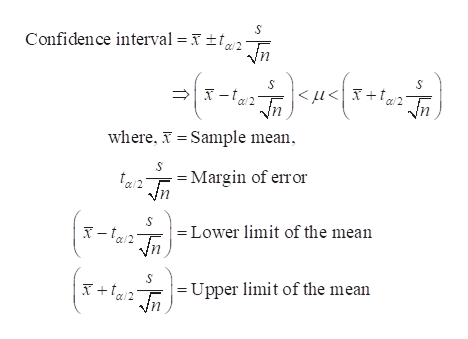# A researcher measured BMI in 12 patients who are free of diabetes and participating in a study of risk factors for obesity and found a mean BMI of 31.7 with a standard deviation of 5.9. Body mass index is measured as the ratio of weight in kilograms to height in meters squared. Generate a two-sided 95% confidence interval estimate of the true BMI. You MUST show your work to receive full credit.

Question
88 views

A researcher measured BMI in 12 patients who are free of diabetes and participating in a study of risk factors for obesity and found a mean BMI of 31.7 with a standard deviation of 5.9. Body mass index is measured as the ratio of weight in kilograms to height in meters squared. Generate a two-sided 95% confidence interval estimate of the true BMI. You MUST show your work to receive full credit.

check_circle

star
star
star
star
star
1 Rating
Step 1

Confidence interval when population standard deviation is not known:

The formula of confidence interval for the population mean when population standard deviation is not given is as follows.help_outlineImage TranscriptioncloseConfidence interval = x f2 Tn < fu<| X +[a2 _n where, Sample mean Margin of error = Lower limit of the mean = Upper limit of the mean fullscreen
Step 2

Computing the 95% confidence interval for population mean:

Here, the sample mean (x-bar) is 31.7.

The sample standard deviation (s) is 5.9.

The confidence level is 0.95.

Thus, the level of significance, α is 0.05 (= 1 – 0.95).

The degrees of freedom (n − 1) is 11 (=12 − 1).

For 95% confidence interval, the cri...

### Want to see the full answer?

See Solution

#### Want to see this answer and more?

Solutions are written by subject experts who are available 24/7. Questions are typically answered within 1 hour.*

See Solution
*Response times may vary by subject and question.
Tagged in

### Statistics